• 文章目录线性空间线性空间的基本性质线性相关、线性无关线性相关，线性无关性质向量组的极大线性无关组的概念基维数参考 线性空间 即向量空间 定义1\large\color{magenta}{\boxed{\color{brown}{定义1} }}定义...
文章目录线性空间线性空间的基本性质线性相关、线性无关线性相关，线性无关性质向量组的秩与极大线性无关组的概念基和维数参考

线性空间01——线性空间、线性相关、线性无关、基和维数、极大线性无关
线性空间02——坐标、坐标变换与基变换、过度矩阵
线性空间03——子空间、子空间的交与和、生成子空间、 子空间的维数公式
线性空间04——子空间的直和、n个子空间的直和、直和分解、直和补
线性空间05——列空间和零空间、维数
线性空间06——行空间和左零空间
线性空间07——四个基本子空间的基与维数_

线性空间
即向量空间
$\large\color{magenta}{\boxed{\color{brown}{定义1} }}$  设 $F$ 是一个数域, $V$ 是一个非空集合.在 $V$ 上定义一个加法运算, 即对于 $V$ 中任意元素 $\alpha, \beta,$ 按照某一法则,在 $V$ 中存在唯一元素与之对应, 记为 $\alpha+\beta,$ 称为 $\alpha$ 与 $\beta$ 的 $\large\color{#70f3ff}{\boxed{\color{green}{加法}}}$  .
$\large\color{magenta}{\boxed{\color{brown}{定义2} }}$ $F$ 和 $V$ 的数乘, 即对于 $F$ 中任意数 $c$ 和 $V$ 中任意元素 $\alpha,$ 按照某一法则, 在 $V$ 中存在唯一元素与之对应, 记为 $c \alpha,$ 称为 $c$ 与 $\alpha$ 的$\large\color{#70f3ff}{\boxed{\color{green}{数乘}}}$ .
$F$ 是一个数域, $V$ 是一个非空集合,若加法和数乘对于任意 $\alpha, \boldsymbol{\beta}, \gamma \in \boldsymbol{V}, \boldsymbol{c}, \boldsymbol{d} \in \boldsymbol{F},$ 都有
四条加法运算封闭:
(1) 交换律：$\alpha+\beta=\beta+\alpha$
(2) 结合律: $(\alpha+\beta)+\gamma=\alpha+(\beta+\gamma)$
(3) 有零元:在 $V$ 中存在元素0, 使对任意 $\alpha \in V,$ 有 $\alpha+\mathbf{0}=\alpha$
(4) 有负元: 对于$V$中每个元素 $\alpha,$ 存在 $\beta\in V,$ 使 $\alpha+\beta=\mathbf{0}$
两条数乘运算封闭：
(5) $(c d) \alpha=c(d \alpha)$
(6) $1 \alpha=\alpha$
两条加法与数乘结合的规则
(7) 数对元素分配律: $c(\alpha+\beta)=c \alpha+c \beta$
(8) 元素对数分配律:$(c+d) \alpha=c \alpha+d \alpha$
则称 $V$ 是 $F$ 上的$\large\color{#70f3ff}{\boxed{\color{green}{线性空间}}}$ $, V$ 中元素称为$\large\color{#70f3ff}{\boxed{\color{green}{向量}}}$.线性空间也称为向量空间.
凡满足以上八条规则的加法及数量乘法也称为线性运算.
$\Large\color{violet}{例1}$
(1) 数域 $F$ 上 $n$ 维列向量全体 $F^{n}$ 对于向量的加法和数乘是线性空间;——向量空间
(2) 数域 $F$ 上 $m \times n$ 矩阵全体 $F^{m \times n}$ 对于矩阵的加法和数乘构成线性空间;——矩阵空间
(3) 数域 $F$ 是数域 $F$ 上的线性空间, 其中加法运算为数的加法, 数乘运算为数的乘法;
(4) $\mathbb{C}$是 $\mathbb{R}$ 上 $n$ 维列向量全体 $C^{n}$ 对于向量的加法和数乘是线性空间;——复向量空间
非空：包含 0
对加法与 $\mathbb{Q}$ -数乘封闭: $\quad \forall \alpha, \beta \in \mathbb{C}, k \in \mathbb{Q}:$ 显然 $\alpha+\beta, \boldsymbol{k} \alpha \in \mathbb{C}$
复数的加法显然含有零元0, 满足交换律, 结合律, 每个复数都有负元
$\forall \alpha, \beta \in \mathbb{C}, \boldsymbol{k}, \boldsymbol{l} \in \mathbb{Q} \quad$ 因为 $\alpha, \beta, \boldsymbol{k}, l$ 都是复数, 显然满足如下等式:
$\begin{array}{ll} \mathbf{1} \cdot \alpha=\alpha & \boldsymbol{k} \cdot(\boldsymbol{l} \cdot \alpha)=\boldsymbol{k} \boldsymbol{l} \cdot \alpha \\ (\boldsymbol{k}+\boldsymbol{l}) \cdot \alpha=\boldsymbol{k} \bullet \alpha+\boldsymbol{l} \bullet \alpha & \boldsymbol{k} \cdot(\alpha+\beta)=\boldsymbol{k} \bullet \alpha+\boldsymbol{k} \bullet \beta \end{array}$
(5) 设 $C[a, b]$ 是实数域 $\mathbb{R}$ 上闭区间 $[a, b]$ 上全体连续函数的集合, 则 $C[a, b]$ 对于函数的加法和数乘构成 $\mathbb{R}$ 上的线性空间.——函数空间
非空: 包含 0 函数
对加法与 $\mathbb{R}$ -数乘封闭: 连续函数之和, 其实数倍仍为连续函数
推广: 某固定区间上黎曼可积(处处可导)的全体实函数类似构成$\mathbb R$-空间
(6)数域 $P$ 上一元多项式环 $F[x]$, 按通常的多项式加法和数与多项式的乘法，构成一个数域 $F$ 上的线性空间.如果只考虑其中次数小于 $n$ 的多项式，再添上零多项式也构成数域 $F$ 上的一个线性空间，用 $F[x]_{n}$ 表示.——多项式空间
线性空间的基本性质
(1) $V$的零向量唯一.
(2) $V$ 中每一个向量的负向量唯一.
(3) 若 $\alpha+\beta=\alpha+\gamma,$ 则 $\beta=\gamma$.
(4) $0 \alpha=0$
(5) $c \mathbf{0}=\mathbf{0}$
(6) $(-1) \alpha=-\alpha$.
(7) $c \alpha=0,$ 则或 $c=0$ 或 $\alpha=0 .$
$\Large\color{violet}{例2}$   记 $V=\{0\},$ 定义加法 $0+0=0,$ 数乘 $c 0=0 .$则 $V$ 是一个线性空间, 称为$\large\color{#70f3ff}{\boxed{\color{green}{零空间}}}$, 记为 $0 .$
$\Large\color{violet}{例3}$   设 $A$ 是数域 $F$ 上的 $m \times n$ 矩阵, 齐次线性方程组 $A X=0$ 的解的全体对于向量的加法和数乘构成F上的线性空间, 称为$A X=0$ 的$\large\color{#70f3ff}{\boxed{\color{green}{解空间}}}$.
线性相关、线性无关
$\large\color{magenta}{\boxed{\color{brown}{定义3} }}$   设 $V$ 是$F$上的线性空间, $\alpha_{1}, \alpha_{2}, \cdots, \alpha_{s}, \beta \in V$ ,若存在 $a_{1}, a_{2}, \cdots, a_{s} \in F,$ 使得
$\beta=a_{1} \alpha_{1}+a_{2} \alpha_{2}+\cdots+a_{s} \alpha_{s}$
则称 $\beta$ 是 $\alpha_{1}, \alpha_{2}, \cdots, \alpha_{s}$ 的$\large\color{#70f3ff}{\boxed{\color{green}{线性组合}}}$, 或称 $\beta$ 可由 $\alpha_{1}, \alpha_{2}, \cdots, \alpha_{s}$ $\large\color{#70f3ff}{\boxed{\color{green}{线性表出}}}$.
$\large\color{magenta}{\boxed{\color{brown}{定义4} }}$  设线性空间 $V$ 中有向量组 $\alpha_{1}, \alpha_{2}, \cdots, \alpha_{s}$ 和$\beta_{1}, \beta_{2}, \cdots, \beta_{t} .$ 若 $\alpha_{1}, \alpha_{2}, \cdots, \alpha_{s}$ 中的每个向量
可由 $\beta_{1}, \beta_{2}, \cdots, \beta_{t}$ 线性表出, 则称向量组$\alpha_{1}, \alpha_{2}, \cdots, \alpha_{s}$ 可由向量组 $\beta_{1}, \beta_{2}, \cdots, \beta_{t}$  $\large\color{#70f3ff}{\boxed{\color{green}{线性表出}}}$ $;$
如果向量组 $\alpha_{1}, \alpha_{2}, \cdots, \alpha_{s}$ 和向量组 $\beta_{1}, \beta_{2}, \cdots, \beta_{t}$可以互相表出, 则称向量组 $\alpha_{1}, \alpha_{2}, \cdots, \alpha_{s}$ 和 $\beta_{1}, \beta_{2}, \cdots, \beta_{t}$  $\large\color{#70f3ff}{\boxed{\color{green}{等价}}}$.
$\large\color{magenta}{\boxed{\color{brown}{定义5} }}$  设 $V$ 是数域 $F$ 上线性空间, $\alpha_{1}, \alpha_{2}, \cdots, \alpha_{s}$ 是 $V$ 中 $s$ 个向量, 若存在不全为零的数 $a_{1}, a_{2}, \cdots, a_{s},$ 使 $a_{1} \alpha_{1}+a_{2} \alpha_{2}+\cdots+a_{s} \alpha_{s}=0,$ 则称 $\alpha_{1}, \alpha_{2}, \cdots, \alpha_{s}$$\large\color{#70f3ff}{\boxed{\color{green}{线性相关}}}$.
若 $\alpha_{1}, \alpha_{2}, \cdots, \alpha_{s}$ 不是线性相关, 则称为$\large\color{#70f3ff}{\boxed{\color{green}{线性无关}}}$. 等价的说法是: 向量组$\alpha_{1}, \alpha_{2}, \cdots, \alpha_{s}$ 称为线性无关, 若存在 $a_{1}, a_{2}, \cdots, a_{s} \in F,$ 使得$a_{1} \alpha_{1}+a_{2} \alpha_{2}+\cdots+a_{s} \alpha_{s}=0,$ 则必有 $a_{i}=0(i=1,2, \cdots, s)$.
线性相关，线性无关性质

设在 $V$ 中 $\beta=a_{1} \alpha_{1}+a_{2} \alpha_{2}+\cdots+a_{s} \alpha_{s},$ 则表示法唯一的充分必要条件是 $\alpha_{1}, \alpha_{2}, \cdots, \alpha_{s}$ 线性无关.

单个向量 $\alpha$ 线性相关 $\Leftrightarrow \alpha=0$；
设 $\alpha_{1}, \alpha_{2}, \cdots, \alpha_{s} \in V$ 且 $s \geq 2,$ 则 $\alpha_{1}, \alpha_{2}, \cdots, \alpha_{s}$ 线性相关的充分必要条件是其中至少有一个向量是其余向量的线性组合.

若 $\alpha_{1}, \alpha_{2}, \cdots, \alpha_{s}$ 是 $V$ 中线性相关的向量组, 则任一包含这组向量的向量组必线性相关.

在 $V$ 中, 若 $\alpha_{1}, \alpha_{2}, \cdots, \alpha_{s}$ 线性无关, $\alpha_{1}, \alpha_{2}, \cdots, \alpha_{s}, \beta$ 线性相关, 则 $\beta$ 可由 $\alpha_{1}, \alpha_{2}, \cdots, \alpha_{s}$ 线性表出且表
示法唯一.

如果向量组 $\alpha_{1}, \alpha_{2}, \cdots, \alpha_{s}$ 可以由向量组 $\beta_{1}, \beta_{2}, \cdots, \beta_{t}$ 线性表出, 且 $s>t,$ 则 $\alpha_{1}, \alpha_{2}, \cdots, \alpha_{s}$ 必然线性相关.

若向量组 $\alpha_{1}, \alpha_{2}, \cdots, \alpha_{r}$ 线性无关，且可被向量组$\beta_{1}, \beta_{2}, \cdots, \beta_{s}$ 线性表出, 则 $r \leq s ;$
若 $\alpha_{1}, \alpha_{2}, \cdots, \alpha_{r}$ 与 $\beta_{1}, \beta_{2}, \cdots, \beta_{s}$ 为两线性无关的等价向量组,则 $r=S .$

向量组的等价关系满足

(1) 【反身性】 即 $\alpha_{1}, \alpha_{2}, \cdots, \alpha_{s}$ 与自身等价.
(2) 【对称性】 即若 $\alpha_{1}, \alpha_{2}, \cdots, \alpha_{s}$ 与 $\beta_{1}, \beta_{2}, \cdots, \beta_{t}$ 等价,则 $\beta_{1}, \beta_{2}, \cdots, \beta_{t}$ 与 $\alpha_{1}, \alpha_{2}, \cdots, \alpha_{s}$ 等价.
(3) 【传递性 】即若 $\alpha_{1}, \alpha_{2}, \cdots, \alpha_{s}$ 与 $\beta_{1}, \beta_{2}, \cdots, \beta_{t}$ 等价,$\beta_{1}, \beta_{2}, \cdots, \beta_{t}$ 与 $\gamma_{1}, \gamma_{2}, \cdots, \gamma_{r}$ 等价, 则 $\alpha_{1}, \alpha_{2}, \cdots, \alpha_{s}$与 $\gamma_{1}, \gamma_{2}, \cdots, \gamma_{r}$ 等价.
向量组的秩与极大线性无关组的概念
如果向量组 $V$ 的部分向量组 $\alpha_{1}, \alpha_{2}, \ldots, \alpha_{r}$ 满足:
(1) $\alpha_{1}, \alpha_{2}, \ldots, \alpha_{r}$ 线性无关;
(2) $V$ 中任意 $r+1$ 个向量都线性相关.
称 $\alpha_{1}, \ldots, \alpha_{r}$ 是 $V$ 的一个 极大无关组，数 $r$ 称为 $V$ 的秩.
约定：只含零向量的向量组的秩为0.
性质:
(1) 等价的无关组含向量个数相同.
(2) 向量组与它的极大线性无关组等价.
(3) 向量组的任意两个极大线性无关组所含元素个数相等.
(4) 向量组线性无关 $\Leftrightarrow$ 秩等于它所含元素的个 数.
(5) 组 $I$ 由组 $II$ 线性表出 $\Rightarrow$ 秩 $I$ $\leq$秩 $II$.
基和维数
$\large\color{magenta}{\boxed{\color{brown}{定义1} }}$  设 $V$ 是数域 $F$ 上非零线性空间, 如果 $V$ 中存在 $n$ 个向量 $\xi_{1}, \xi_{2}, \cdots, \xi_{n},$ 满足:
$(1) \xi_{1}, \xi_{2}, \cdots, \xi_{n}$ 线性无关;
(2) $V$ 中任意向量可表示为 $\xi_{1}, \xi_{2}, \cdots, \xi_{n}$ 的线性组合;
则称 $\xi_{1}, \xi_{2}, \cdots, \xi_{n}$ 是 $V$ 的一个基. 若 $V$ 中有 $n$ 个向量为$\large\color{#70f3ff}{\boxed{\color{green}{基}}}$ $,$ 则称 $V$ 为 $n$ $\large\color{#70f3ff}{\boxed{\color{green}{维线性空间}}}$, 并记为 $\operatorname{dim}_{F} V=n$ 或 $\operatorname{dim} V=n .$
定义零空间的维数为0. 不是有限维的线性空间称为$\large\color{#70f3ff}{\boxed{\color{green}{无限维线性空间}}}$.
$\Large\color{violet}{注 }$： $n$ 维线性空间 $V$ 的基不是唯一的, $V$中任意 $n$ 个线性无关的向量都是V的一组基.
$\Large\color{violet}{注 }$： $n$ 维线性空间 $V$ 的任意两组基向量是等价的.
$\Large\color{violet}{注 }$ : 基就是 $V$ 的一个极大无关组, 维数就是 $V$ 的秩.
$\Large\color{violet}{例1}$： $P^{n}=\left\{\left(a_{1}, a_{2}, \cdots, a_{n}\right) \mid a_{i} \in P, i=1,2, \cdots, n\right\}$ 为 $n$ 维的 $,$
$\varepsilon_{1}=(1,0, \cdots, 0), \varepsilon_{2}=(0,1, \cdots, 0), \cdots, \varepsilon_{n}=(0, \cdots, 0,1)$
就是 $P^{n}$ 的一组基. 称为 $P^{n}$ 的标准基.
$\large\color{magenta}{\boxed{\color{brown}{定义2} }}$  若在线性空间 $V$ 中有 $n$ 个线性无关的向量, 但是任意 $n+1$ 个向量都是线性相关的, 则称 $V$ 是一个$n$ 维线性空间; 常记作 $\operatorname{dim} V=n .$
$\large\color{magenta}{\boxed{\color{brown}{定义3} }}$  若线性空间 $V$ 中可以找到任意多个线性无关的向量,则称 $V$ 是无限维线性空间.
$\Large\color{violet}{例2}$： 所有实系数多项式所成的线性空间 $R[x]$ 是无限维的, 因为 :对任意的正整数 $n,$ 都有 $n$ 个线性无关的向量
$1, x, x^{2}, \ldots, x^{n-1}$ 。
$\Large\color{violet}{例3}$    $n$ 维单位列向量 $\varepsilon_{1}, \varepsilon_{2}, \cdots, \varepsilon_{n}$ 是 $F^{n}$ 的一个基,所以 $\operatorname{dim} F^{n}=n$
$\Large\color{violet}{例4}$   数域 $\mathbb{C}$ 作为 $\mathbb{C}$ 上的线性空间 $,$ 它的维数
$\operatorname{dim}_{\mathbb{C}} \mathbb{C}=1$
数1 就是一组基,1 是 $\mathbb{C}$ -线性无关的: $\quad$ 设 $k \in \mathbb{C}$ 使得 $\boldsymbol{k} \cdot 1=\mathbf{0}$, 则 $\boldsymbol{k}=\mathbf{0}$.
任一 $c \in \mathbb{C}$ 均可由 1 用复系数线性表出: $\quad c=c \cdot 1$.
(2) 将 $\mathbb{C}$ 看成 $\mathbb{R}$ 一线性空间,则 $\operatorname{dim}_{\mathbb{R}}(\mathbb{C})=\mathbf{2}$, 数 $1, \mathrm{i}$ 是一组基.
$1, \mathrm{i}$ 是 $\mathbb{R}$ -线性无关的: 设 $\boldsymbol{a}, \boldsymbol{b} \in \mathbb{R}$ 使得 $\boldsymbol{a} \cdot 1+\boldsymbol{b} \cdot \mathrm{i}=\mathbf{0}$, 则 $\boldsymbol{a}=\boldsymbol{b}=\mathbf{0} .$
任一 $a+b \mathbf{i} \in \mathbb{C}(a, b \in \mathbb{R})$ 均可由 $1, \mathbf{i}$ 用实系数线性表出: $\quad a+b \mathbf{i}=a \cdot 1+b \cdot \mathbf{i}$
$\Large\color{violet}{例5}$   证明数域 $F$ 上的基础矩阵 $E_{i j}(i=1,2, \cdots, m ;$ $j= 1,2, \cdots, n)$ 是 $F^{m \times n}$ 的一个基.
【证明】 设 $\quad \sum_{i=1}^{m} \sum_{j=1}^{n} c_{i j} E_{i j}=0$ ，其中 $c_{i j} \in F(i=1,2, \cdots, m ; j=1,2, \cdots, n)$，因为 $\quad 0=\sum_{i=1}^{m} \sum_{j=1}^{n} c_{i j} E_{i j}=\left(c_{i j}\right)_{m \times n}$。其中 $c_{i j}=0(i=1,2, \cdots, m ; j=1,2, \cdots, n)$ 。
这就证明了 $E_{i j}(i=1,2, \cdots, m, j=1,2, \cdots, n)$ 线性无关.
另一方面, 对于任意的 $A=\left(a_{i j}\right)_{m \times n},$ 有
$A=\sum_{i=1}^{m} \sum_{j=1}^{n} a_{i j} E_{i j}$
这就说明了 $F^{m \times n}$ 中任意矩阵可以由基础矩阵 $E_{i j}(i=1,2, \cdots, m ; j=1,2, \cdots, n)$ 线性表出。所以 $E_{i j}(i=1,2, \cdots, m ; j=1,2, \cdots, n)$ 是$F^{m \times n}$ 的一个基， 它的维数
$\operatorname{dim} F^{m \times n}=m n$
参考
高等代数，林亚南，高等教育出版社
高等代数学习辅导，林亚南，林鹭，杜妮，陈清华，高等教育出版社
高等代数    电子科技大学
高等代数_安阳师范学院
《高等代数》（第五版）


展开全文数学建模 数据挖掘 机器学习 深度学习
• 就是矩阵中 极大线性无关组的向量个数。 也就是 以这个矩阵的元素作为系数的方程组中，线性无关的方程个数。 线性无关，就是 a，b，c个方程中，谁也不能通过自己或者另外两个方程表示谁。 线性相关线性无关...
秩

矩阵的秩。秩 就是矩阵中 极大线性无关组的向量个数。

也就是 以这个矩阵的元素作为系数的方程组中，线性无关的方程个数。

线性无关，就是 a，b，c个方程中，谁也不能通过自己或者另外两个方程表示谁。

线性相关和线性无关：

线性相关的意思就是若有一组向量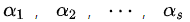，能有一组不全为0的常数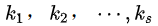，使得：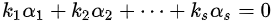，则表示，为[线性相关]的向量。

反之，若要使成立，只能是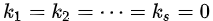的话，那么就表示这组向量[线性无关]。

简单来说就是，在线性相关的向量里，里面每一个向量都可以被其他向量来表示。线性无关的向量，则是里面的向量无法通过其他向量来表示。那这有什么用呢？以下列方程组为例：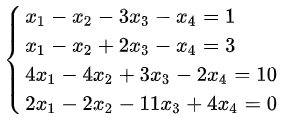能否解出唯一解呢？上过初中的人都知道，4条方程，4个未知数，能解出唯一解。但是！！前提是这四条方程必须是线性无关的。为什么呢？你想想看，如果第一条方程可以通过其余三条表示的话，那意味着，其余三条方程也可以构造出其他方程，那不就变成一生二二生三，三生万物..几条方程可以构造无限方程吗？那就很耍赖啊，要是你通过几条方程变来变去构造出50条方程的话，那难道就能解50个未知数的方程了吗？这显然不行的，所以构造的方程都很水的，只是同样的一些方程，换了衣服而已。所以要解四个未知数的方程组，必须要四条线性无关的方程，其中这四条方程谁也不能表示谁，即谁也不同通过线性变化变成谁。

如上面的方程，如果你将第一个方程的-1、-4、-2倍分别加在随后的各个方程上，再变换一下，就可以得到：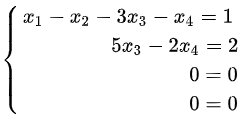看到没有，后面的两组方程，0=0，说的是废话，所以后面两条方程并没有包含任何信息，是多余的。真正有意义的只有前两条方程。这两个有意义的方程的系数，组成2个系数的向量，这两个向量就是整个方程组的 [极大线性无关组] ，方程组内的所有向量都可以由 这个 [极大线性无关组] 表示。

而 秩 就是 方程组内 极大线性无关组 的向量个数。

那你可能又问了，秩不是矩阵的秩吗？你说的是方程组啊。。。。

方程组的常数系数就是矩阵中的数字。

所以 秩 的大小，表示了这个矩阵所散发出的信息量的多少。


展开全文• 文章目录极大线性无关组定理向量组的(与矩阵的定义完全不同)定理行与列...任何一个极大线性无关组和其向量组可以相互表示，即等价 定理 这个部分组是极大线性无关组的条件是： 极大无关组不唯一，但是任意...
文章目录极大线性无关组定理向量组的秩(与矩阵的秩定义完全不同)定理行秩与列秩定理例题参考

极大线性无关组
一个向量组用极大无关组就可以表示剩余所有向量。极大无关组是所含线性无关向量最多的一个。全是0的向量组没有极大线性无关组
线性无关的向量组的极大线性无关组是其本身
任何一个极大线性无关组和其向量组可以相互表示，即等价
定理
这个部分组是极大线性无关组的条件是：极大无关组不唯一，但是任意两个极大无关组所含的向量个数是相同的

向量组的秩(与矩阵的秩定义完全不同)
极大无关组含有向量的个数是几则向量组的秩就是几定理特别的，若这两个向量组等价则这两个向量组的秩相等。

行秩与列秩
一个矩阵，行向量组组的秩为行秩，列向量组的秩叫做列秩。
定理：矩阵的行秩一定等于列秩，并且等于矩阵的秩。求向量组的秩一般转化为求矩阵的秩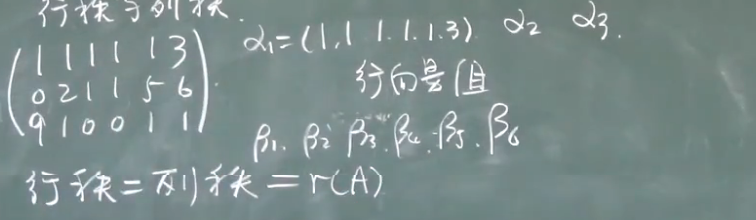定理例题
下面的例题是求一个向量组的极大无关组参考
以上图片均摘自宋浩老师视频，以方便以后自己查阅，感谢宋老师。
视频传送门


展开全文• 本篇笔记首介绍了矩阵的行和列，...最后重点介绍了极大线性无关组的求法，根据矩阵的初等行（列）变换不改变其列（行）向量间的线性关系，将矩阵化为行简化阶梯形，然后直接写出极大线性无关组和其向量的线性表示。
本篇笔记首介绍了矩阵的行秩和列秩，即矩阵的行秩等于矩阵的列秩等于矩阵的秩，而且矩阵乘积的秩不大于每个因子的秩；还介绍了求矩阵行秩和列秩的方法，即化为阶梯形矩阵；最后重点介绍了极大线性无关组的求法，根据矩阵的初等行（列）变换不改变其列（行）向量间的线性关系，将矩阵化为行简化阶梯形，然后直接写出极大线性无关组和其向量的线性表示。
1 矩阵的行秩和列秩
举例：矩阵$A=\begin{bmatrix}1&1&1&1&1&3\\0&2&1&1&5&6\\9&1&0&0&1&1\end{bmatrix}$，
取出所有的行形成行向量，
$\alpha_1=\begin{pmatrix}1&1&1&1&1&3\end{pmatrix}$，
$\alpha_2=\begin{pmatrix}0&2&1&1&5&6\end{pmatrix}$，
$\alpha_3=\begin{pmatrix}9&1&0&0&1&1\end{pmatrix}$，
全体$\alpha_1,\alpha_2,\alpha_3$称为矩阵$A$的行向量组。
取出所有的列形成列向量，
$\beta_1=\begin{pmatrix}1\\0\\9\end{pmatrix},\beta_2=\begin{pmatrix}1\\2\\1\end{pmatrix},\beta_3=\begin{pmatrix}1\\1\\0\end{pmatrix}$，
$\beta_4=\begin{pmatrix}1\\1\\0\end{pmatrix},\beta_5=\begin{pmatrix}1\\5\\1\end{pmatrix},\beta_6=\begin{pmatrix}3\\6\\1\end{pmatrix}$，
全体$\beta_1,\beta_2,\beta_3,\beta_4,\beta_5,\beta_6$称为矩阵$A$的列向量组。
定义：矩阵行$\underline{向量组的秩}$称为矩阵的行秩，矩阵$\underline{列向量组的秩}$称为矩阵的列秩。
上述例子中，行向量有$3$个，行向量组的秩最大为$3$；而列向量有$6$个，列向量组的秩最大为$6$。那么矩阵的行秩和列秩会不会不一样呢？
答案是否定的。
因为列向量虽然有$6$个，但它是$3$维的，所以秩最大也是$3$。
那么矩阵的行秩和列秩是否相等呢？
定理3.3.4：对任何矩阵$A$，均有：$\color{red}{A的行秩=A的列秩=r(A)}$。
矩阵的秩是用非零子式定义的，而向量组的秩是用极大无关组定义的，定义方式完全不同，但最终竟然相等！
举例：矩阵$A=\begin{bmatrix}\color{red}{1}&\color{red}{0}&0&0\\\color{red}{0}&\color{red}{1}&0&0\\0&0&0&0\\0&0&0&0\end{bmatrix}$，
其最高阶数的非0子式为$\begin{bmatrix}1&0\\0&1\end{bmatrix}$，
所以$r(A)=2$；
很明显要找它的行秩或列秩，那么就先找到其极大无关组，如
使用$\beta_1=\begin{pmatrix}1\\0\\0\\0\end{pmatrix},\beta_2=\begin{pmatrix}0\\1\\0\\0\end{pmatrix}$可以表示所有的列向量，如
$\beta_1=1\times\beta_1+0\times\beta_2+0\times\beta_3+0\times\beta_4$，
$\beta_2=0\times\beta_1+1\times\beta_2+0\times\beta_3+0\times\beta_4$，
$\beta_3=0\times\beta_1+0\times\beta_2+0\times\beta_3+0\times\beta_4$，
$\beta_4=0\times\beta_1+0\times\beta_2+0\times\beta_3+0\times\beta_4$，
可以看出，这两列是“最重要”且不可缺少的，用这两列就可以表示其他所有的列，
而这两个列向量$\beta_1,\beta_2$就是矩阵$A$列向量组$\beta_1,\beta_3,\beta_1,\beta_4$的极大无关组，
所以$r(\beta_1,\beta_3,\beta_1,\beta_4)=2$，
即矩阵$A的列秩为2$，
故$A的列秩=r(A)$，
同理可得，$A的行秩=r(A)$，
故$A的行秩=A的列秩=r(A)$。
定理3.3.5：（考研用的多）矩阵乘积的秩不大于每个因子的秩。
即：$\color{red}{r(AB){\le}min\{r(A),r(B)\}}$，
显然，可以推广到有限个矩阵相乘的情形上去：
$\color{red}{r(A_1A_2...A_n){\le}\underset{1{\le}i{\le}n}{min}r(A_i)}$。
2 求秩举例
例1：求矩阵$A=\begin{bmatrix}3&3&3\\2&-1&5\\-5&3&-13\\4&-3&11\end{bmatrix}$的行秩和列秩。
解：使用初等行变换化为阶梯形，
$A=\begin{bmatrix}3&3&3\\2&-1&5\\-5&3&-13\\4&-3&11\end{bmatrix}\xrightarrow[]{初等行变换}\begin{bmatrix}1&1&1\\0&-3&3\\0&0&0\\0&0&0\end{bmatrix}$，
由此可见$r(A)=2$，所以矩阵$A$的行秩和列秩都是$2$。
例2：求向量组$\alpha_1=(1,-2,2,-1),\alpha_2=(2,-4,8,0),\alpha_3=(-2,4,-2,3),\alpha_4=(3,-6,0,-6)$的秩，并判断是否线性相关。
解：以$\alpha_1^T,\alpha_2^T,\alpha_3^T,\alpha_4^T$为列向量构成矩阵$A$，并将矩阵$A$实施初等行变换，化为阶梯形矩阵，
$A=\begin{bmatrix}1&2&-2&3\\-2&-4&4&-6\\2&8&-2&0\\-1&0&3&-6\end{bmatrix}\xrightarrow[]{初等行变换}\begin{bmatrix}1&2&-2&3\\0&2&1&-3\\0&0&0&0\\0&0&0&0\end{bmatrix}$
显然，$r(A)=2$，所以$r(\alpha_1,\alpha_2,\alpha_3,\alpha_4)=2<4$，
故$\alpha_1,\alpha_2,\alpha_3,\alpha_4$线性相关。
3 求极大无关组
定理3.3.6：若对$m{\times}n$的矩阵$A$仅实施初等行变换化为矩阵$B$，那么矩阵$A$的列向量组$\alpha_1,\alpha_2,...,\alpha_n$同矩阵$B$的列向量组$\beta_1,\beta_2,...,\beta_n$有完全相同的线性关系。
以上可以简述为：矩阵的初等行（列）变换不改变其列（行）向量间的线性关系。
举例：$A=\begin{bmatrix}1&0&5\\0&1&3\\0&0&0\end{bmatrix}$，
若将矩阵$A$实施初等行变换得到$B$矩阵，即
$A=\begin{bmatrix}1&0&5\\0&1&3\\0&0&0\end{bmatrix}\xrightarrow[将第二行加到第三行]{将第一行加到第三行}\begin{bmatrix}1&0&5\\0&1&3\\1&1&8\end{bmatrix}=B$，
矩阵$A$的列向量组为：
$\alpha_1=\begin{pmatrix}1\\0\\0\end{pmatrix},\alpha_2=\begin{pmatrix}0\\1\\0\end{pmatrix},\alpha_3=\begin{pmatrix}5\\3\\0\end{pmatrix}$，
矩阵$B$的列向量组为：
$\beta_1=\begin{pmatrix}1\\0\\1\end{pmatrix},\beta_2=\begin{pmatrix}0\\1\\1\end{pmatrix},\beta_3=\begin{pmatrix}5\\3\\8\end{pmatrix}$，
很明显$\alpha_1,\alpha_2$线性无关，而$\beta_1,\beta_2$是$\alpha_1,\alpha_2$的接长向量，故$\beta_1,\beta_2$也是线性无关，
再看$\alpha_3=5\alpha_1+3\alpha_2$，同时$\beta_3=5\beta_1+3\beta_2$，
故矩阵的初等行（列）变换不改变其列（行）向量间的线性关系。
$\color{red}{★★★}$ 例3.3.3：求向量组$\alpha_1=(1,-2,2,-1),\alpha_2=(2,-4,8,0),\alpha_3=(-2,4,-2,3),\alpha_4=(3,-6,0,-6)$的一个极大无关组，并给出其余向量用该极大无关组的线性表示。
解：
① 不管是行向量还是列向量，均将向量组按列构成矩阵
$A=\begin{bmatrix}1&2&-2&3\\-2&-4&4&-6\\2&8&-2&0\\-1&0&3&-6\end{bmatrix}$，
② 只用初等行变换化成行简化阶梯形
$\xrightarrow[]{只做初等行变换}\begin{bmatrix}1&0&-3&6\\0&1&\frac{1}{2}&-\frac{3}{2}\\0&0&0&0\\0&0&0&0\end{bmatrix}$，
③ 首非零元所在的列做极大无关组；
$\beta_1=\begin{pmatrix}1\\0\\0\\0\end{pmatrix},\beta_2=\begin{pmatrix}0\\1\\0\\0\end{pmatrix}$是极大线性无关组，
④ 其余向量表示系数直接写出来
$\beta_3=-3\beta_1+\frac{1}{2}\beta_2$，
$\beta_4=6\beta_1-\frac{3}{2}\beta_2$，
⑤ 写出$\alpha$的极大无关组和线性表示
由定理3.3.6可知，$\alpha_1,\alpha_2$是$\alpha_1,\alpha_2,\alpha_3,\alpha_4$的一个极大无关线，而且
$\alpha_3=-3\alpha_1+\frac{1}{2}\alpha_2$，
$\alpha_4=6\alpha_1-\frac{3}{2}\alpha_2$，
注：第③④可以省略。
举例：如果最后化成的行简化阶梯形为$\begin{bmatrix}1&0&0&0&1\\0&1&3&0&1\\0&0&0&1&2\\0&0&0&0&0\end{bmatrix}$，
$\begin{bmatrix}&&\alpha_3&&\alpha_5\\&&\downarrow&&\downarrow\\1&0&0&0&1\\0&1&3&0&1\\0&0&0&1&2\\0&0&0&0&0\\\uparrow&\uparrow&&\uparrow&\\\alpha_1&\alpha_2&&\alpha_4&\end{bmatrix}$,
所以$\alpha_1,\alpha_2,\alpha_4$是极大无关组，
$\alpha_3=0\alpha_1+3\alpha_2+0\alpha_4$，
$\alpha_5=\alpha_1+\alpha_2+2\alpha_4$。
常见错误：没有化成行简化阶梯形，如只化成阶梯形。
举例：$\begin{bmatrix}1&1&2&1\\0&1&0&1\\0&0&0&0\end{bmatrix}$，
$\alpha_1,\alpha_2$是极大无关组，
$\alpha_3=?$
$\alpha_4=?$
4 引用
《线性代数》高清教学视频 “惊叹号”系列 宋浩老师_哔哩哔哩 (゜-゜)つロ 干杯~-bilibili_3.3 向量组的秩（二）


展开全文• 1、向量组bai的线性代du数的基本概念，它表示的是一个zhi向量组的极大线dao性无关组所含向量的个数。由向量组的可以引出矩阵的的定义。 2、矩阵的线性代数中的一个概念。在线性代数中，一个矩阵A的列...
• 本篇笔记首先回顾了矩阵的，然后通过两个例子引入了极大线性无关组的定义，并由极大无关组定义介绍了一些结论，还介绍了相关定理；然后给出了向量组的定义，以及一些结论定理；向量组的与矩阵的，定义方式...
• 向量组的轶指的是极大线性无关组中向量的个数 矩阵的轶是把一个矩阵分为行向量组和列向量组,这两个向量组的轶分别称为行轶和列轶.可以证明的是行轶和列轶相等,这就是矩阵的轶. 这里提醒一下就是: n-r为线性无关的解...
• 1. 向量组的 设有向量组(α1,α2...........αn​)其中所含有的极大线性无关组的个数就是向量组的 例:考虑向量组α1(1,0,0),α2(0,1,0),α3(0,0,1),α4(1,1,0),α5(1,1,1)\alpha_1(1,0,0),\alpha_2(0,1,0),...
• 矩阵论，线性代数中的rank：极大线性无关组所含向量的个数。 举例子： 对于二维数组（矩阵）A： A = [[1, 0, 0], [0, 1, 0], [0, 0, 1]] numpy：rank = 2(其是二维数组) 矩阵论，线性代数：rank=3 （极大线性...
• 学习机器学习, 基础的线性代数知识是必备的基础功, 对于线性代数的探索, 向量组也是线性代数的重要基础. 今天我们就开始学习一下线性代数...向量组的极大无关组 2.1 极大无关组 2.2 等价向量组 向量空间 3.1
• 求他们的极大线性无关组, 也就是括住的向量组的 , (基是极大线性无关组) 两个等价的线性无关向量组含有的相同个数的向量 线性变换
• ## 秩 (线性代数)

千次阅读 2011-04-01 11:18:00
在线性代数中，一个矩阵A的列是A的线性无关的纵列的极大数目。类似地，行是A的线性无关的横行的极大数目。矩阵的列秩和总是相等的，因此它们可以简单地称作矩阵A的。通常表示为r(A)，rk(A)或rank A。m ...matrix system 算法 c
• 线性代数 - 04 向量的线性关系 ...二、极大线性无关组与向量组的 1、极大线性无关组的概念 2、极大线性无关组的求法 3、向量组的 转载于:https://www.cnblogs.com/haicheng/p/3701548.html...
• 引出： 看到上面的方程，我们会想到那些问题： 1、这是一个n元一次方程组，小时候老师经常说，n元方程想要解出n未知数，需要n的方程组才可以得出解，后来发现...则称该部分组是这个向量组的极大线性无关组。..
• 本章四大问题：线性表出、线性相关、极大线性无关组、等价向量组 矩阵相乘本质上还是可以看成向量的内积： 引言总结： 就是线性无关的向量个数（不含有多余的信息） 矩阵的秩和向量组的本质相同 线性相关...
• 行列式 定义 性质 展开定理 几个重要的行列式 用行或列表示的行列式的性质 ...极大线性无关组，等价向量组，向量组的 向量空间 线性方程组 齐次线性方程组 非齐次线性方程组 特征值与特征向...
• 行列式的本质： 是N维多边体的体积。 矩阵的：  极大线性无关组的求法：  初等行变换 矩阵的迹： 1.等于对角线元素之。 2.等于特征值之
• 每个向量有权值，求最小权极大线性无关组 本题是使用贪心策略的高斯消元 由输入给出的n个物品，每个物品有m种属性，价格price 如果a物品的属性可以由其他已有物品的属性组合出，那么a可以不必购买 问最.....
• 向量间的线性关系 线性相关 存在不全为0的解 ...极大线性无关组 向量组中，无关向量数最多的一组（可以有多组） 向量组的：极大无关组含有的向量个数 矩阵的行秩和 定理：矩阵的 行=列=r(A) ...
• 目录一、向量及其线性运算二、向量组的线性相关三、线性相关的判定定理四、线性相关判定定理45的证明五、向量组的极大无关组的定义六、向量组的极大无关组的求法七、向量空间八、向量的正交性九、向量组...
• 向量组的极大无关组和秩 向量组的秩与矩阵的秩 线性方程组的解的结构 解的存在性与唯一性 齐次线性方程组的基础解系 非齐次线性方程组的一般解 在解析几何中的应用 向量空间 Rn的子空间 基和维数 坐标和...
• 文章目录三、向量1 向量的定义运算1.1 定义1.2 运算2 线性组合与线性表示2.1 线性组合2.2 线性表示2.3 向量组的等价3 线性相关与线性无关3.1 定义3.2 性质4 向量组的4.1 向量组的极大线性无关组4.2 向量组的5 ...考研数学
• 利用线性无关定义“极大线性无关组” 定理 b可被一组向量线性表出 一组向量a线性相关 部分组和整体组 延伸组和缩短组 s个向量线性相关 s个向量线性不关，s+1个向量线性相关 关于两个向量组线性表出的 一直...
• 线性代数》复习提纲 第一部分：基本要求（计算方面） 四阶行列式的计算； ...N阶特殊行列式的计算（如有行、列相等）；...求向量组的极大无关组，并将多余向量用极大无关组线性表示； 将无..
• 标量：只有大小没有方向的物理量 向量：又称矢量，既包含大小又包含方向的物理量 矩阵：矩阵是一个二维数组，其中的每一个元素...矩阵的：矩阵列向量中的极大线性无关组的数目，记作列。行=列=矩阵的...机器学习
• 设A是一组向量，定义A的极大无关组中向量的个数为A的。 矩阵A的列是A的线性独立的纵列的极大数目，通常表示为r(A)或rank A。 满：m × n矩阵的为mn中的较小者。（设A是n阶矩阵, 若r（A） = n, 则称A为满...
• dim(O)=0 例子 基础解系的个数=矩阵的维数−矩阵的基础解系的个数=矩阵的维数-矩阵的基础解系的个数=矩阵的维数−矩阵的 ...零空间是找基础解系，列空间是找系数矩阵（列向量构成的极大线性无关组）。 ...矩阵论
• 线性代数核心问题求多元方程的解。核心技能乘积、内积、已知矩阵 A 矩阵 B，求 A B 的乘积 C=AB。...在线代中的定义：一个矩阵 A 的列是 A 的线性无关的列的极大数目。类似地，行...
• 对线代的各章节内容分别给出清晰的网络图，对于行列式，矩阵，向量组的秩和极大无关组，齐次与非齐次方程组的解法，向量空间，特征值与特征向量相互之间的关系，以及它们的求解方法也给出了总结。内容全面，学习简单...
• 否则向量就是线性无关的。 ：在线性代数中，一个矩阵A的列是A的线性獨立的纵列的极大数目。类似地，行是A的线性獨立的横行的极大数目。 矩阵的列秩和总是相等的，因此它们可以简单地称作矩阵A的。...